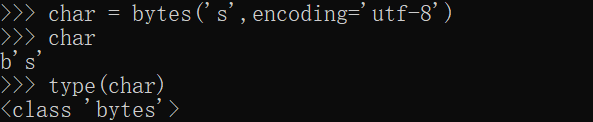# 天空洒雨会长风

## 微雨是露空迷眼 直指剑河莫知倦 未见长空多疑路 何须再会梦长风——————————脩于公元二零一九年五月二十六日

## 【python你不知道的知识】如何实现python中的字符（注意不是字符串）

`char = bytes('s',encoding='utf-8')`## 【mysql技巧】mysql中查询一个字段属于什么表什么数据库

```select table_schema,table_name from information_schema.columns where
column_name = '字段名'```

## 风中冥

### 老僧呆闺中心游百里槐闲庭坐风里盘膝九曲愁

——————————脩于公元二零一九年三月二十五日

## 一、C++11的新功能：

### 2.自动类型推断关键字auto和decltype()得到类型

#### ①使用auto进行自动类型推导：

```//auto的使用示例
//auto tmp; // 错误，auto是通过初始化表达式进行类型推导，如果没有初始化表达式，就无法确定tmp的类型
auto tmp = 1;//等价于int tmp = 1;
auto tmp = 1.0; //等价于 double tmp = 1.0;
auto tmp = "Hello World";  //等价于 char *tmp = "Hello World";
auto tmp  = 'A';  //等价于 char tmp  = 'A';
auto func = less<int>();
vector<int> arr;
auto it = arr.begin();  //等价于vector<int>::iterator it   = arr.begin();
auto p = new foo(); // 对自定义类型进行类型推导```

```//auto应用于模版
//1.不使用auto
template <class Student, class Teacher>
void levelUp(const Teacher& th)
{
}

//2.使用auto
template <class Teacher>
void levelUp(const Teacher& th)
{
}```

#### ②使用decltype()得到类型：

```//使用decltype(）得到变量类型
int x = 3;
decltype(x) y = x;

template <class Teacher>
void levelUp(const Teacher& th)->decltype(th.levelPlus();）
{
}```

### 3.使用默认构造函数和删除（default：A()=default; A()=delete;）

```//默认构造函数和删除

### 4.匿名函数lambda表达式

lambda表达式类似Javascript中的闭包，它可以用于创建并定义匿名的函数对象，以简化编程工作。

Lambda语法：[函数对象参数]（操作符重载函数参数）->返回值类型{函数体}

```//lambda表达式
vector<int> arr{5, 4, 3, 2, 1};
int a = 2, b = 1;

for_each(arr.begin(), arr.end(), [b](int &x){cout<<(x + b)<<endl;});
//b就是指函数可以得到在Lambda表达式外的全局变量
for_each(arr.begin(), arr.end(), [=](int &x){x *= (a + b);});
//在[]中传入=的话，即是可以取得所有的外部变量
for_each(arr.begin(), arr.end(), [=](int &x)->int{return x * (a + b);});

//①[]内的参数指的是Lambda表达式可以取得的全局变量
//②()内的参数是每次调用函数时传入的参数
//③->后接的是Lambda表达式返回值的类型```

（中第23②）

### 6.右值引用T&&与移动构造函数：

##### c.移动构造函数为什么使用右值引用:

```//（1）声明一个右值引用
int&& i = 42;

class X
{
private:
int* data;
public:X():data(new int){}~X(){delete [] data;}
X(const X& other):data(new int){std::copy(other.data,other.data+1000000,data);}
//拷贝构造函数
X(X&& other):data(other.data){other.data=nullptr;}};
//移动构造函数

//（2）使用在拷贝构造函数
X x1;
X x2 = std::move(x1);
X x3 = static_cast<X&&>(x2);

//（3）使用在函数模板
//填入左值，推断为左值引用;填入右值，推断为普通无修饰类型。
foo(42);foo(3.14159);foo(std::string());
//填入右值，推断为普通无修饰类型情况
int i=42;foo(i);
//填入左值，推断为左值引用情况```

### 7.初始化列表(更加优雅的初始化方法)：

```//初始化列表(更加优雅的初始化方法)

//引入C++11之前，只有数组能使用初始化列表，
//其他容器想要使用初始化列表，只能用以下方法:
int arr = {1, 2, 3}
vector<int> arr(arr, arr + 3);

//C++11中，我们可以使用以下语法来进行替换：
int arr{1, 2, 3};
vector<int> arr{1, 2, 3};
map<int, string>{{1, "a"}, {2, "b"}};
string str{"Hello World"};```

### 8.加长参数模板：

```//加长参数模板

//在C语言中printf可以传入多个参数，在C++11中，
//可以用加长参数模板实现更简洁的Print

Print(tail...);
}```

### 9.元组tuple：

```//C++中的pair可以使用make_pair构造，构造一个包含两种不同类型的数据的容器
auto p = make_pair(1, "C++ 11");

//C++11中引入了变长参数模板(参见8)，所以发明了新的数据类型：tuple
//tuple是一个N元组，可以传入1个， 2个甚至多个不同类型的数据
auto t1 = make_tuple(1, 2.0, "C++ 11");
auto t2 = make_tuple(1, 2.0, "C++ 11", {1, 0, 2});
```

### 10.序列for循环：

```//C++中for循环可以使用类似java的简化的for循环，
//可以用于遍历数组，容器，string以及由begin和end函数定义的序列
map<string, int> m{{"a", 1}, {"b", 2}, {"c", 3}};
for (auto p : m){
cout<<p.first<<" : "<<p.second<<endl;
}```

c++11部分参考了文章：https://www.cnblogs.com/guxuanqing/p/6707824.html

## 二、C++14加入的新功能：

### 1.Lambda函数在C++11的基础上更加方便：

```//C++11要求Lambda参数使用具体的类型声明
auto lambda = [](int x, int y) {return x + y;};

//C++14的泛型Lambda使编写如下语句成为可能：
auto lambda = [](auto x, auto y) {return x + y;};

//新标准中的std::move函数可用于捕获Lambda表达式中的变量，
//这是通过移动对象而非复制或引用对象实现的：
std::unique_ptr ptr(new int(10));
auto lambda = [value = std::move(ptr)] {return *value;};```

### 3.类型推导:

##### C++11仅支持Lambda函数的类型推导，C++14对其加以扩展，支持所有函数的返回类型推导： auto DeducedReturnTypeFunction(); 因为C++14是强类型语言，有些限制需要考虑：

①如果一个函数的实现中有多个返回语句，这些语句一定要推导出同样的类型。

②返回类型推导可以用在前向声明中，但是在使用它们之前，翻译单元中必须能够得到函数定义。

③返回类型推导可以用在递归函数中，但是递归调用必须以至少一个返回语句作为先导，以便编译器推导出返回类型。

## 猿类面试小书（C++,游戏，unity方向,后台）

3.一个linux内核相关的项目TKeed：

https://github.com/linw7/TKeed

## C++面试常考基础（C++常考基础知识）

（由修根据深信服等公司的流出面试题和各公司的面经总结）

### 1.C++基本类型和占用的存储空间：

#### 数值范围：

 类型 比特数 有效数字 数值范围 float 32 6~7 -3.4*10(-38)～3.4*10(38) double 64 15~16 -1.7*10(-308)～1.7*10(308) long double 128 18~19 -1.2*10(-4932)～1.2*10(4932)

### 3.C++中new和C中的malloc的区别（尼禄、zoom）：

#### ③返回类型：

##### new操作符内存分配成功时，返回的是对象类型的指针，类型严格与对象匹配，无须进行类型转换，故new是复合类型安全的操作符。而malloc内存分配成功则是返回void*，需要通过强制类型转换将void*指针转换成我们需要的类型。例如：
`buffer=（char*)malloc(i+1);`

### 5.C++中哪些机制可以替代宏定义常量：

#### ①const替换宏

(1) 当定义的是常量指针，需要const两次（ const char* const iVar = “C++”）,第一个const是防止值被改变，第二个const防止地址被改变。
(2) 当定义到class内时，需要用static来修饰这个变量，防止出现多个实体。

### 6.C++在new失败后的处理方法：

#### ①C++标准做法

```try
{
double *ptr=new double;
}
{
//失败以后，要么abort要么重分配
cerr<<memExp.what()<<endl;
}
```

#### ② 分配内存的时候创建一个指针，判断是否是空指针

```double *ptr=new double;
if( 0 == ptr)
```

### 7.某函数希望既可以被C调用，又可以被C++调用，应该怎么声明它的原型？

#### C++语言支持函数重载，C 语言不支持函数重载。函数被C++编译后在库中的名字与C 语言的不同。假设某个函数的原型为： void foo(int x, int y);该函数被C 编译器编译后在库中的名字为_foo ， 而C++ 编译器则会产生像_foo_int_int 之类的名字。 C++提供了C 连接交换指定符号extern“C”来解决名字匹配问题。

```/*TestC.h*/
#ifndef TESTC_H
#define TESTC_H

#ifdef __cplusplus
extern "C"{
#endif
int add(int a , int b);

#ifdef __cplusplus
}
#endif

#endif```

```/*TestC.c*/
#include"TestC.h"

int add(int a , int b)
{
return(a+b);
}
/*TestC.cc*/
#include <iostream>
#include "TestC.h"
int main()
{
cout << add(2,5) << endl ;
return 0;
}```

#### 用一个函数将C++类的使用封装起来，然后将它外部声明为C函数就可以了。

```/* ADD.h */
{
public:
{
return(a+b);
}
};

//*将C++类封装为C函数文件

extern "C" int add_cpp(int a , int b);

int add(int a , int b)
{
}

//*实际调用C++代码的C文件

extern int add_cpp(int a , int b);

int main()
{
return 0;
}

```

### 9.举例说明explicit关键字的用法：

#### C++中的explicit关键字只能用于修饰构造函数, 它的作用是表明该构造函数是显式的, 而非隐式的, 跟它相对应的另一个关键字是implicit, 意思是隐藏的,类构造函数默认情况下即声明为implicit(隐式)。explicit关键字的作用就是防止类构造函数的隐式自动转换。

```//A使用隐式转换implicit
class A
{
public:
A(int){}
A(int,int){}
operator bool const {return true;}
};
//B类型使用显式转换explicit
class B
{
public:
explicit B(int){}
explicit B(int,int){}
explicit operator bool const {return true;}
};

int main()
{
A a1 = 1 ; //OK,使用A::A(int)
A a2(2); //OK,使用A::A(int)
A a3{4,5};//OK,使用A::A(int,int);
A a4 ={4,5};//OK,使用A::A(int,int);
A a5 =  (A)1;//OK,使用 static_cast
bool na1 = a1 ;//OK,复制构造函数使用 A::operator bool()
bool na2 = static_cast<bool>(a1);//OK  使用static_cast 直接赋值

B b1 = 1 ; //出错,不会使用B::B(int)
B b2(2); //OK,使用B::B(int)
B b3{4,5};//OK,使用B::B(int,int);
B b4 ={4,5};//出错,不会使用B::B(int,int);
B b5 =  (B)1;//OK,使用 stbtic_cbst
bool nb1 = b1 ;//出错,不会使用 B::operbtor bool()
bool nb2 = stbtic_cbst<bool>(b1);//OK  使用stbtic_cbst 直接赋值
}```

### 13.写出重载前++和后++的函数声明：

#### ①前++：

`self & operator++();`

#### ②后++：

`self operator++(T);//T是类型名，如int`

### 16.C++中如何禁止子类默认的拷贝函数，默认构造拷贝函数不会被执行？

```class T{
T(const T&) = delete; //禁用复制构造函数
T(T&&) = default;   //启用默认的移动构造函数
};
```

### 25.C++单例模式：

#### 通过单例模式可以保证类只有一个实例,并提供一个访问它的全局访问点,该实例被所有程序模块共享。 单例模式的要点有三个：a. 单例类只能有一个实例 b. 它必须自行创建这个实例 c. 它必须自行向整个系统提供提供这个实例 优点：减少了时间和空间的开销，提高了封装性。 具体实现：将构造函数、复制构造函数、析构函数和重载赋值号=等设为私有，禁止重载；提供一个静态的函数接口以供外部调用。

```class SingleSample
{
public:
static SingleSample * getInterface();
private:
SingleSample();
SingleSample(const SingleSample &);
SingleSample & operator=(const SingleSample &);
~SingleSample();

static SingleSample * interface ;
}

//初始化：
SingleSample * SingleSample::interface;
SingleSample * SingleSample::getInterface()
{
return interface;
}```

### 27.strlen()和sizeof()的区别（zoom面试）：

#### （3）举例：

```	char s[] ="hello";
char *p = s;
//注意 \0 不算在内
cout << strlen(s) << endl;//5
cout << strlen(p) << endl;//5
cout << sizeof(s) << endl;//6
cout << sizeof(p) << endl;//4```

### 28.C++继承中重载、重写、重定义的区别（zoom面试）：

#### 本文地址：

（由修根据深信服等公司的流出面试题和各公司的面经总结）

## 惊破天

#### 铁骑横飞踏破天

——————————脩于公元二零一八年七月二十二日夜

## windows 下配置mysql-5.7.21（最新免安装版）

（注：由于软件时境过迁，只保证2018.4.10日（今天）此方法可用，后续软件升级后可能也会有些许出入）

1、新建一个变量：MYSQL_HOME

2、修改path变量

 12345678910111213141516171819202122232425262728293031323334353637 [mysqld] #绑定IPv4 bind-address = 0.0.0.0 # 设置mysql的安装目录,即你解压缩安装包的位置 basedir = D:/soft/mysql # 设置mysql数据库的数据的存放目录 datadir = D:/soft/mysql/data # 设置端口号 port = 3306 # 允许最大连接数 max_connections = 200 # 设置字符集为utf8 loose-default-character-set = utf8 # 开启查询缓存 explicit_defaults_for_timestamp = true # windows下区分表名大小写(0:不区分，2区分(5.7版本)) lower_case_table_names=2 sql_mode=NO_ENGINE_SUBSTITUTION,STRICT_TRANS_TABLES [client] #设置客户端字符集 port=3306 default-character-set = utf8 [WinMySQLadmin] Server = D:/soft/mysql/bin/mysqld.exe skip-grant-tables

1、使用管路员权限打开CMD（注意管理员权限），在CMD中进入mysql的解压目录

2、运行服务安装命令

mysqld install MySQL –defaults-file=”%MYSQL_HOME%\my.ini”

1、启动服务命令为：net start mysql
2、此时会提示：

①首先打开注册表
win+r打开运行，输入regedit，打开注册表
②更改注册表：找到
HKEY_LOCAL_MACHINE
-SYSTEM
-CurrentControlSet
-services-mysql(服务名)
-ImagePath

2、打开管理工具 服务，找到MySQL服务。通过右键选择启动或者直接点击左边的启动来启动服务

 1 mysqld --initialize-insecure --user=mysql

 1 mysql -uroot -p